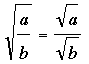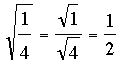SEARCH HOMEMath Central Quandaries & QueriesQuestion from Lauren, a student: How do you solve square roots of fractions? Does the format change if it is either a proper or an improper fraction? Ex. the square root of 1/4 or the square roots of 80/25Lauren,

You can use the fact thatsoFor 80/25 you can apply the same technique or you could notice that 5 divides the numerator and denominator and hence write 80/25 = 16/5 before taking the square root of the numerator and denominator.

I hope this helps,
PennyMath Central is supported by the University of Regina and The Pacific Institute for the Mathematical Sciences.